# Contraction semi-group

A one-parameter strongly-continuous semi-group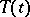,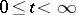,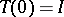, of linear operators in a Banach spacefor which. An operatorthat is densely defined inis a generating operator (generator) of the contraction semi-group if and only if the Hille–Yosida condition is satisfied: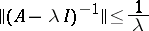for all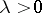. In other words, a densely-defined operatoris a generator of a contraction semi-group if and only if is a maximal dissipative operator.

Contraction semi-groups in Hilbert space have been studied in detail. Particular forms of contraction semi-groups are semi-groups of isometries, unitary semi-groups, self-adjoint semi-groups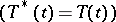and normal semi-groups. Instead of the generatorit is sometimes convenient to use its Cayley transform: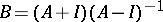(a cogenerator). It turns out that a semi-group is a semi-group of isometries, or a unitary, a self-adjoint, or a normal semi-group if and only if the cogenerator is, respectively, an isometric, a unitary, a self-adjoint, or a normal operator.

A contraction semi-group is called completely non-unitary, if its restriction to any invariant subspace is not unitary. For a completely non-unitary semi-groupas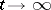, for any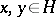. In order that a contraction semi-group is completely non-unitary it is sufficient that it be stable, that is, that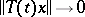as, for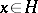.

For every contraction semi-groupthere is an orthogonal decompositioninto-invariant subspaces such that the semi-group is unitary on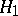and completely non-unitary on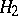.

Ifis a contraction semi-group in a Hilbert space, then there is a larger Hilbert space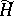, containingas a subspace, and in it a unitary group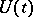,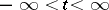, such that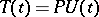for, whereis the orthogonal projection fromonto. The groupis called a unitary dilation of the semi-group. The dilation is uniquely defined up to an isomorphism if it is required thatcoincides with the closed linear span of the set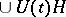() (a minimal dilation).

Letbe a Hilbert space and let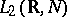be the Hilbert space of all measurable-valued functions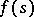,, with square-integrable norm. In this space, the unitary group of two-sided shifts,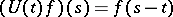, is defined. Similarly, the semi-group of one-sided shifts is defined in the space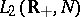,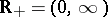;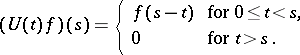Every completely non-unitary semi-group of isometries is isomorphic to the one-sided shift onfor some suitable space.

Ifis a completely non-unitary contraction semi-group andis its minimal unitary dilation, then on some invariant subspace of(but ifis stable, then on the whole of) the group is isomorphic to that of two-sided shifts. For contraction semi-groups with non-linear operators, see Semi-group of non-linear operators.

How to Cite This Entry:
Contraction semi-group. Encyclopedia of Mathematics. URL: http://encyclopediaofmath.org/index.php?title=Contraction_semi-group&oldid=51663
This article was adapted from an original article by S.G. Krein (originator), which appeared in Encyclopedia of Mathematics - ISBN 1402006098. See original article